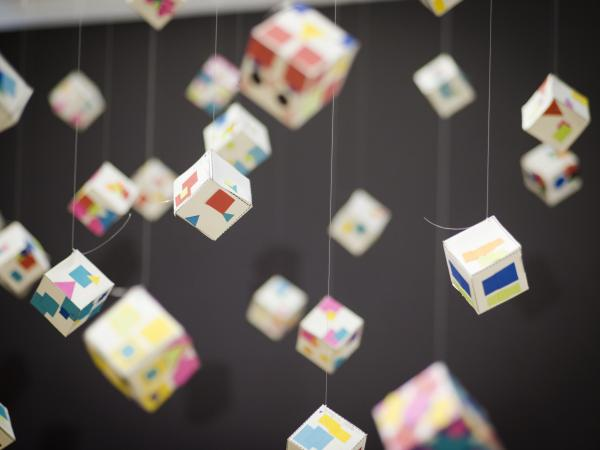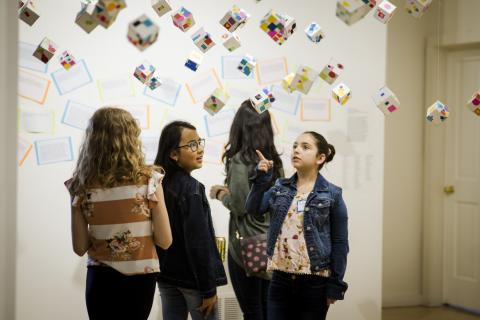# Express Yourself! Math Expressions

March 2, 2020
Image### Students explore how to decompose and recompose equations and how equivalent expressions can be expressed differently.

This graphic design project uses symbols to create various expressions of the same equation on each side of a cube. Symbols represent numerical values, giving students a key from which to base their designs and equations.

### Objectives:

• Students will learn to plan compositions
• Students will develop patterns using symbols
• Students will consider movement, balance, and color in their sculptures
• Students use equivalent expressions to design different patterns on each side of their sculpture
• Students will increase their understanding of equations through the development and creation of their art

### Basic Lesson Outline:

• Introduce the project
• Introduce symbols and demonstrate recording, planning, and forming equivalents
• Students plan and design each side of their sculpture
• Students begin cutting and structuring their sculptures
• On the final workday for sculptures, students begin their written reflection
• Students present their sculpture as a part of a class gallery

### Art Supplies:

• Construction paper
• Shape templates for 3D structures
• Templates for symbols
• Glue
• Scissors
• Envelopes

### Other Resources:

• Example of designed sculpture
• Slide show to introduce project and related artwork (see outline at the end of the lesson plan)### Idaho State Learning Standards

Arts and Humanities: Anchor Standard 4: Convey meaning through the presentation/performance/production of an original work or unique interpretation of a work.

• Objective PR1.1 Combine knowledge and understanding from two or more disciplines to present/perform their original or interpreted works for an audience
• Objective PR1.2 Convey meaning through their presentation/performance

Math 6.EE. Apply and extend previous under standings of arithmetic to algebraic expressions

1. Write and evaluate numerical expressions involving whole-number exponents
2. Apply the properties of operations to generate equivalent expressions. For example, apply the distributive property to the expression 3 (2 + x) to produce the equivalent expression 6 + 3x; apply the distributive property to the expression 24x + 18y to produce the equivalent expression 6 (4x + 3y); apply properties of operations to y + y + y to produce the equivalent expression 3y
3. Identify when two expressions are equivalent (i.e., when the two expressions name the same number regardless of which value is substituted into them). For example, the expressions y + y + y and 3y are equivalent because they name the same number regardless of which number y stands for

• Subject area language: expressions, equivalent
• Art language: sculpture, movement, balance, pattern, symmetry, color, shape, form

### Student Use of Vocabulary:

Students will use the words when creating, writing about, and discussing their projects

### Student Grouping:

Students will work independently